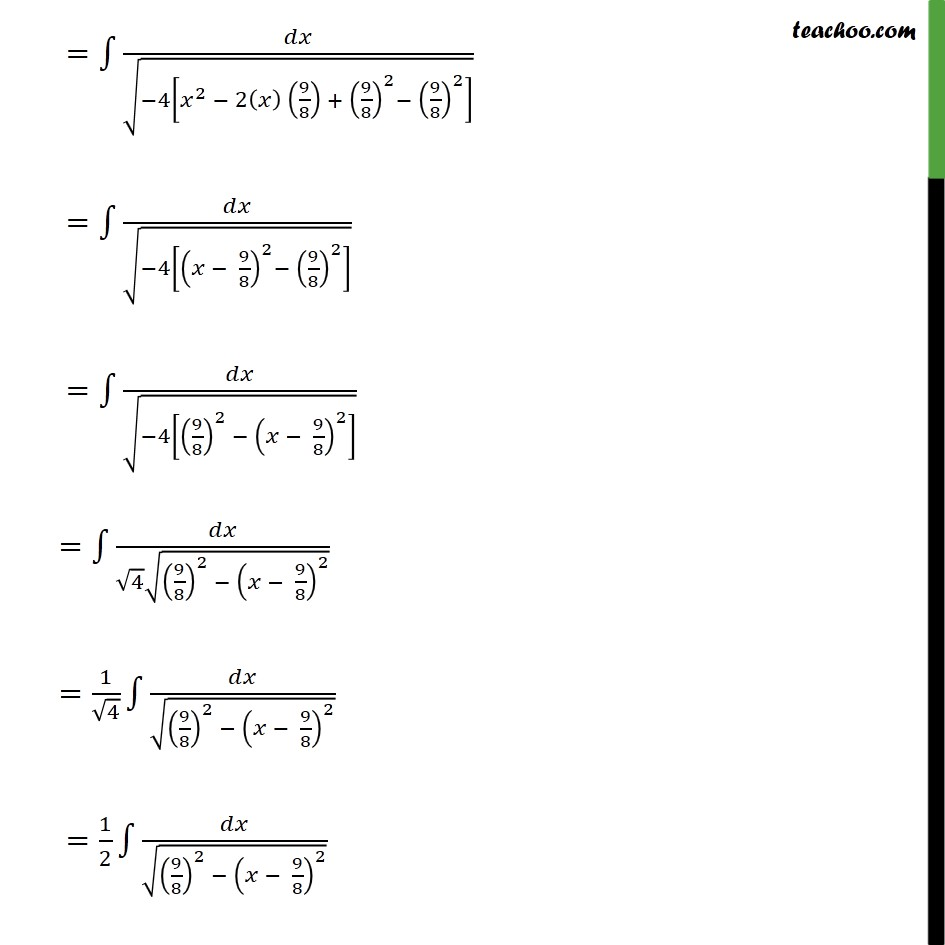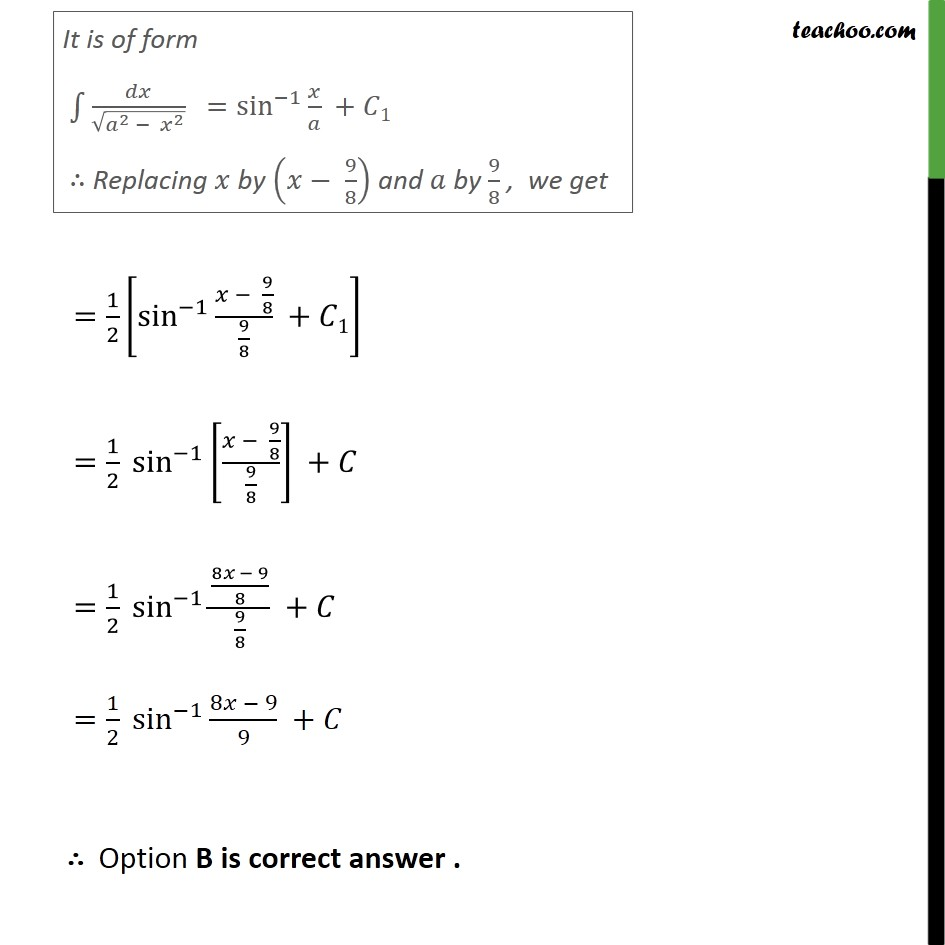1. Chapter 7 Class 12 Integrals
2. Serial order wise
3. Ex 7.4

Transcript

Ex 7.4, 25 9 4 2 equals A. 1 9 sin 1 9 8 8 + C B. 1 9 sin 1 8 9 9 + C C. 1 3 sin 1 9 8 8 + C D. 1 2 sin 1 9 8 8 + C 9 4 2 = 4 2 9 4 = 4 2 2 9 8 = 4 2 2 9 8 + 9 8 2 9 8 2 = 4 9 8 2 9 8 2 = 4 9 8 2 9 8 2 = 4 9 8 2 9 8 2 = 1 4 9 8 2 9 8 2 = 1 2 9 8 2 9 8 2 = 1 2 sin 1 9 8 9 8 + 1 = 1 2 sin 1 9 8 9 8 + = 1 2 sin 1 8 9 8 9 8 + = 1 2 sin 1 8 9 9 + Option B is correct answer .

Ex 7.4

Chapter 7 Class 12 Integrals
Serial order wise